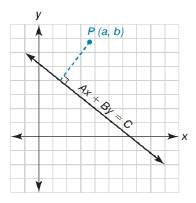Chapter 10.5, Problem 47EElementary Geometry For College St...

7th Edition
Alexander + 2 others
ISBN: 9781337614085

Solutions

Chapter
SectionElementary Geometry For College St...

7th Edition
Alexander + 2 others
ISBN: 9781337614085
Textbook Problem

Describe the steps of the procedure that enables us to find the distance from a point P a ,   b to the line A x + B y = C .To determine

Write the steps of the procedure that enables us to find the distance from a point Pa, b to the line Ax+By=C.Explanation

The steps which enables us to find the diatance from a point to the line is as follows:

Initially find the equation for a line which passes through the point Pa, b and is perpendicular to the line Ax+By=C.

Then solve these two equations in order to find the point of intersection of the lines.

This point of intersection is the another point on the line which passes through the point Pa, b.

Thus the two points are known, we can able to find the distance between the points by using the distance formula

Still sussing out bartleby?

Check out a sample textbook solution.

See a sample solution

The Solution to Your Study Problems

Bartleby provides explanations to thousands of textbook problems written by our experts, many with advanced degrees!

Get Started

Find more solutions based on key concepts